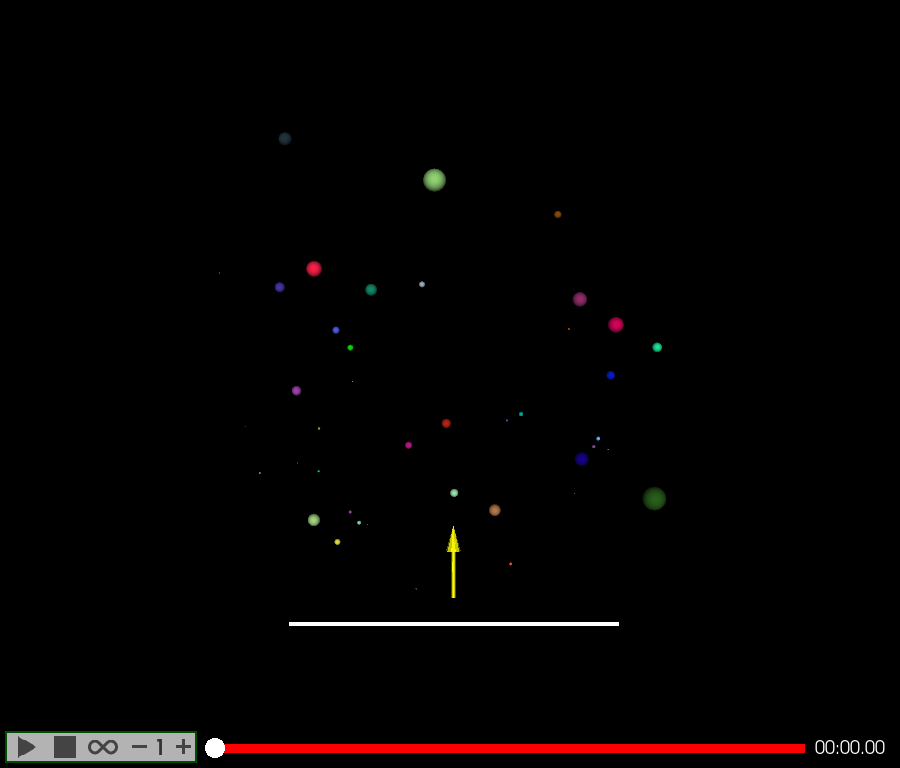# Keyframe animation: Camera and opacity#

Camera and opacity keyframe animation explained in this tutorial.

```import numpy as np
from fury import actor, window
from fury.animation import Timeline, Animation, CameraAnimation
from fury.animation.interpolator import cubic_spline_interpolator
```

## The Plan#

The plan here is to animate (scale and translate) 50 spheres randomly, and show FURY text that appears at the end!

```scene = window.Scene()

showm = window.ShowManager(scene,
size=(900, 768), reset_camera=False,
order_transparent=True)
```

## Creating the main `Timeline` and adding static actors to it#

Here we create a `Timeline`. so that we can use it as a controller for the 50 animations we will create. So, Instead of updating and adding 50 Animations to the `ShowManager`, we only need to update the main `Timeline`. Also, a playback panel can be assigned to this main Timeline.

But, why we need 50 `Animations`, you may ask. -> A single `Animation` can handle each property once at a time. So we need 50 `Animations` to translate and scale our 50 spheres.

`playback_panel=True` assigns a playback panel that can control the playback of its `Animations`

```timeline = Timeline(playback_panel=True)
```

Creating two actors for visualization, and to detect camera’s animations.

```arrow = actor.arrow(np.array([[0, 0, 0]]), np.array([[0, 1, 0]]),
np.array([[1, 1, 0]]), scales=5)
plan = actor.box(np.array([[0, 0, 0]]), colors=np.array([[1, 1, 1]]),
scales=np.array([[20, 0.2, 20]]))
```

## Creating “FURY” text#

```fury_text = actor.vector_text("FURY",
pos=(-4.3, 15, 0),
scale=(2, 2, 2))
```

Creating an `Animation` to animate the opacity of `fury_text`

```text_anim = Animation(fury_text, loop=False)
```

opacity is set to 0 at time 29 and set to one at time 35. Linear interpolator is always used by default.

```text_anim.set_opacity(29, 0)
text_anim.set_opacity(35, 1)
```

`text_anim` contains the text actor is added to the Timeline.

```timeline.add_animation(text_anim)
```

## Creating and animating 50 Spheres#

```for i in range(50):
###########################################################################
# create a sphere actor that's centered at the origin and has random color
actors = [actor.sphere(np.array([[0, 0, 0]]),
np.random.random([1, 3]),
np.random.random([1, 3]))]

###########################################################################
# create a timeline to animate this actor (single actor or list of actors)
animation = Animation(actors)

# We generate random position and scale values from time=0 to time=49 each
# two seconds.
for t in range(0, 50, 2):
#######################################################################
# Position and scale are set to a random value at the timestamps
# mentioned above.
animation.set_position(t,
np.random.random(3) * 30 - np.array(
[15, 0, 15]))
animation.set_scale(t, np.repeat(np.random.random(1), 3))

###########################################################################
# change the position interpolator to cubic spline interpolator.
animation.set_position_interpolator(cubic_spline_interpolator)

###########################################################################
# Finally, the ``Animation`` is added to the ``Timeline``.
```

## Animating the camera#

Since, only one camera is needed, camera animations are preferably done using a seperate `Animation`. Three properties can control the camera’s animation: Position, focal position (referred to by focal), and up-view.

```camera_anim = CameraAnimation(loop=False)
```

Multiple keyframes can be set at once as follows. camera focal positions

```camera_positions = {
# time: camera position
0: np.array([3, 3, 3]),
4: np.array([50, 25, -40]),
7: np.array([-50, 50, -40]),
10: np.array([-25, 25, 20]),
14: np.array([0, 16, 25]),
20: np.array([0, 14.5, 20]),
}

# camera focal positions
camera_focal_positions = {
# time: focal position
15: np.array([0, 0, 0]),
20: np.array([3, 9, 5]),
23: np.array([7, 5, 3]),
25: np.array([-2, 9, -6]),
27: np.array([0, 16, 0]),
31: np.array([0, 14.5, 0]),
}
```

`set_camera_focal` can only set one keyframe, but `set_camera_focal_keyframes` can set a dictionary of keyframes.

```camera_anim.set_focal_keyframes(camera_focal_positions)
camera_anim.set_position_keyframes(camera_positions)
```

Change camera position and focal interpolators

```camera_anim.set_position_interpolator(cubic_spline_interpolator)
camera_anim.set_focal_interpolator(cubic_spline_interpolator)
```

Adding non-animatable actors to the scene.

```scene.add(arrow, plan)
```

Adding the timeline to the ShowManager.

```showm.add_animation(timeline)
```

The ShowManager must go on!

```interactive = False

if interactive:
showm.start()

window.record(scene, out_path='viz_keyframe_animation_camera.png',
size=(900, 768))
```Total running time of the script: ( 0 minutes 0.260 seconds)

Gallery generated by Sphinx-Gallery